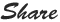# Get Excel Sheet Name - Simple Formula

Here is the formula to get the Excel file name -

=MID(CELL("filename",A1),FIND("]",CELL("filename",A1))+1,31)

Simply put this formula in excel file cell , it will give you a result with sheet name